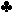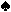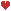J,J,5,3,Q +6,4 = 1.11120605 = One Pair of JacksK,2 = 1.11131205 = One Pair of Jacks8,9 = 1.11120908 = One Pair of Jacks4,K = 1.11131205 = One Pair of JacksJ,5 = 6.1105 = Full House - Jacks over Fives7,7 = 2.110712 = Two Pairs - Jacks and SevensK,6 = 1.11131206 = One Pair of Jacks8,10 = 1.11121008 = One Pair of JacksQ,9 = 2.121109 = Two Pairs - Queens and JacksA,7 = 1.11141207 = One Pair of JacksA,Q = 2.121114 = Two Pairs - Queens and Jacks5,8 = 2.110512 = Two Pairs - Jacks and Fives Winner: Full House - Jacks over Fives (6.1105)

0.0007

6.1105